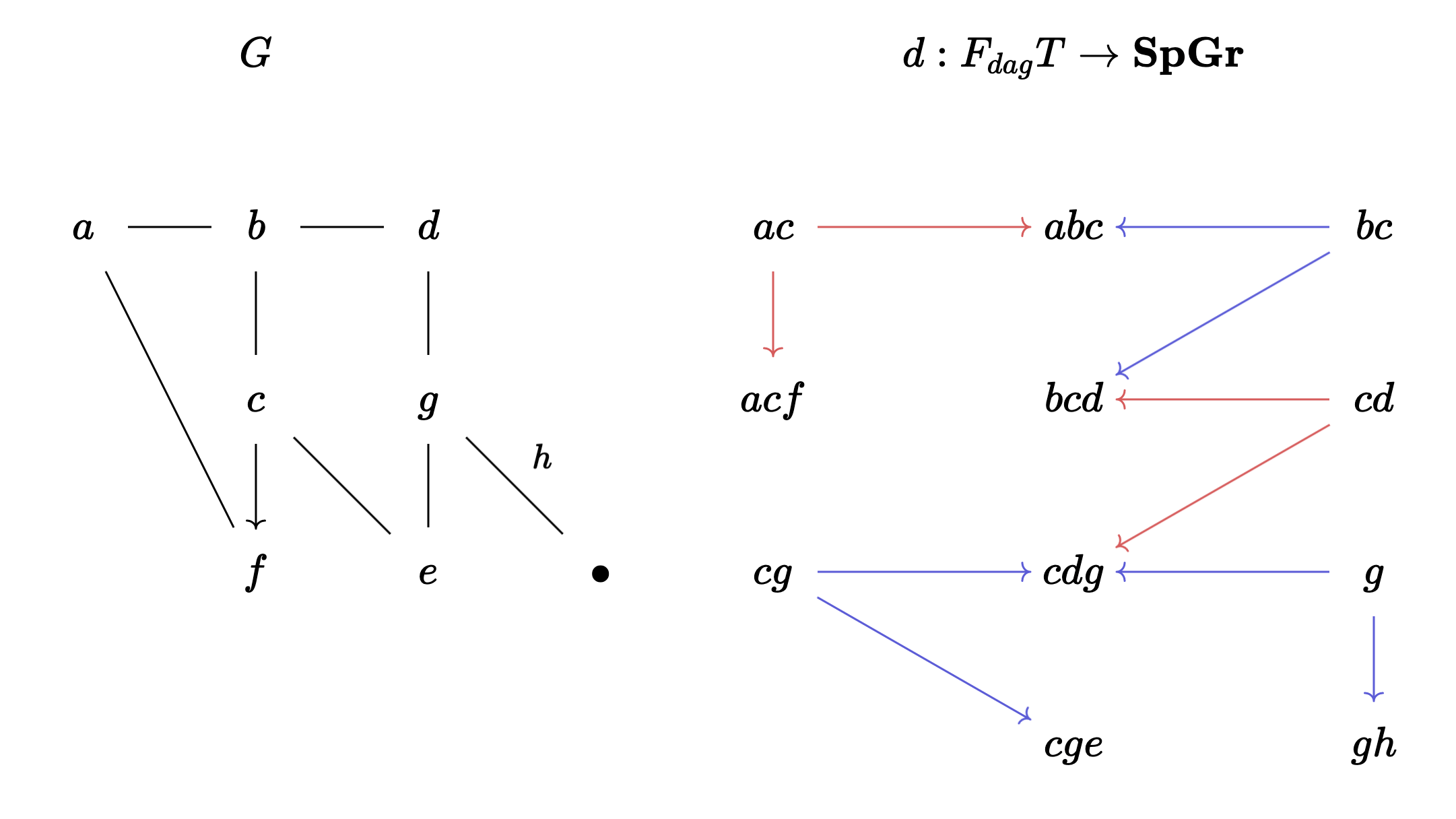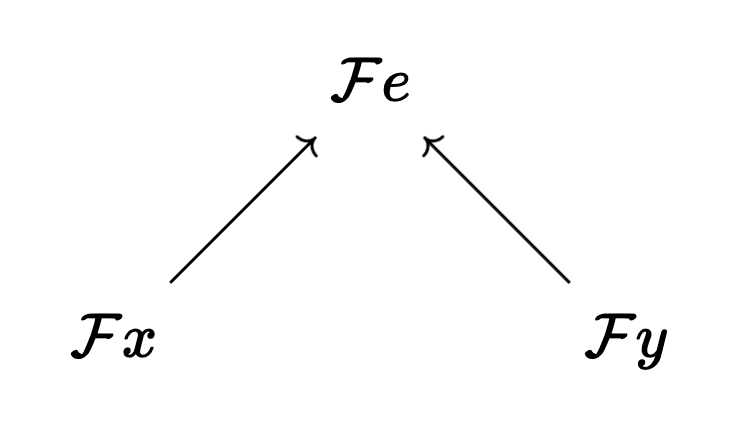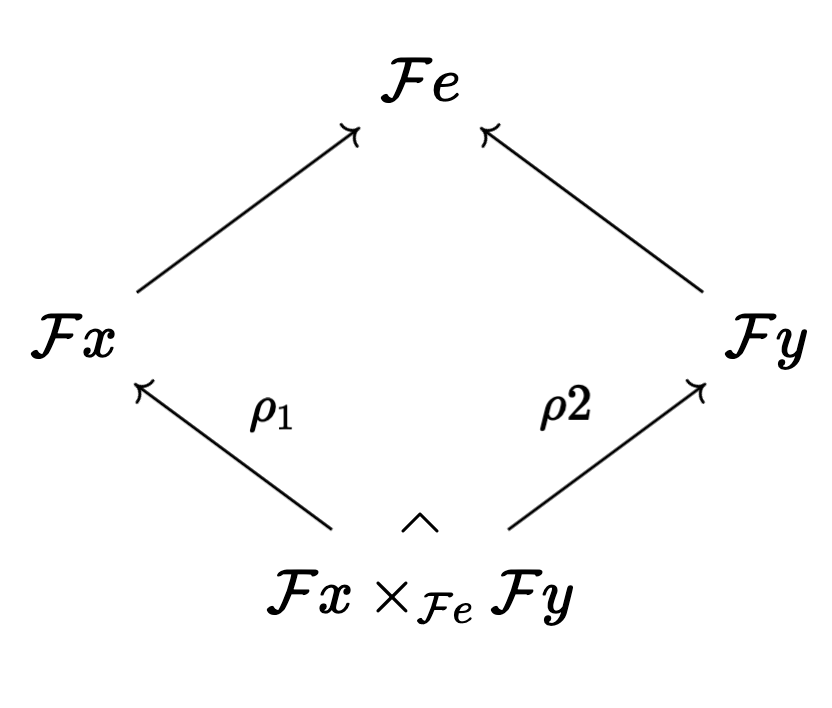# Merlin's Notebook

A blog by Benjamin Merlin Bumpus

# Dynamic programming on non-recursive structures

In a previous post I mentioned a very simple algorithm for solving decision problems encoded as sheaves on inputs that display some recursive structure. In this post I’ll talk about what goes wrong with that approach when we’re trying to compute on inputs that are presented in a recursive, tree-like way and I’ll also propose a route for circumnavigation. But first let’s review what I mean by “recursive structure”.

This post was inspired by conversations with Ernst Althaus, and by conversations with James Fairbanks, and Daniel Rosiak.

### Structured decompositions

In this blog I’ve spoke a lot about structured decompositions. These are ways of building objects (or morphisms) in a category out of smaller and simpler parts. They can be thought of as generalized graphs: a structured decomposition consists of a collection of bags (the simple building blocks) and a collection of adhesions (spans with bags for feet) which act as generalized relations between the bags. Formally, given a graph $$G$$ and a category $$C$$ with pullbacks, a $$C$$-valued structured decomposition of shape $$G$$ is a diagram $$d$$ of the following form $$d: F_{dag}G \to \mathbf{Sp}C$$ where $$F_{dag}G$$ is the free dagger category on $$G$$, $$d$$ is a dagger functor and $$\mathbf{Sp}C$$ is the span category on $$C$$.

Reïterating this for clarity, note that for any vertex $$v$$ of $$G$$, the image $$dv$$ is called a bag while, for any edge $$e=xy$$ in $$G$$, the image $$de$$ (which is a span with feet $$dx$$ and $$dy$$) is called an adhesion.

There is a lot to be said about $$C$$-valued structured decompositions; for example they form a category – denoted $$\mathcal{D}C$$ – and they can be used to recover many useful combinatorial invariants including tree-width, graph decomposition width, layered tree-width, co-tree-width and a refinement of $$\mathcal{H}$$-tree-width. However, for this post all you need to know is how they work for graphs.

A tame graph-valued structured decomposition (which, to avoid being verbose, I’ll simply refer to as a “structured decomposition” in the rest of this post) is a structured decomposition having graphs for bags and monic spans of graphs for adhesions. Here’s a picture of a tree-shaped structured decomposition $$d$$ (right) of a graph $$G$$ (left).The graph $$G$$ on the left is obtained as a colimit of the strucrtured decomposition on the right. Note that some spans are colored red and some are colored blue simply to make the spans easier to see. Each bag and adhesion set above is representing the induced subgraph on those vertices while each leg of the span is the obvious inclusion of graphs.

### Sheaves on tree-shaped structured decompositions

Tree-shaped structured decompositions are among the simplest to compute upon. The tree-shape clearly displays the recursive structure of any graph that is obtained as a colimit of such a decomposition and this structure can be exploited algorithmically. Let’s see how to compute sheaves on such decompositions. (By the way, if you’re new to sheaves, I’ve collected some notes on sheaves in a previous post; you can find it here.)

Suppose we have a sheaf $$\mathcal{F}: (\mathbf{Sub}G)^{op} \to \mathbf{FinSet}$$ which for concreteness you could think of as the $$n$$-coloring sheaf which takes each subgraph $$G’$$ of $$G$$ to the set $$\mathbf{Gr}(G’, K_n)$$ of all homomorphisms from $$G’$$ to $$K_n$$ (note that I’m working with irreflexive graphs here).

We can decide whether this sheaf admits a global section (which in the coloring example above translates to deciding whether $$G$$ is $$n$$-colorable) by using any tree-shaped structured decomposition $$d: F_{dag}T \to \mathbf{Sp}\mathbf{Gr}$$ as follows.

Algorithm 1. Fix any ordering $$e_1, \dots, e_m$$ of the edges of $$T$$. For each edge $$e=xy$$ proceed as follows:

• consider the associated adhesion• under the sheaf $$\mathcal{F)$$ this gives us a cospan• take the pullbackof this cospan and then replace $$\mathcal{F}x$$ and $$\mathcal{F}y$$ by the images of the pullback under the projections $$\rho_1$$ and $$\rho_2$$.

To see why this works for coloring, let’s first unpack what this algorithm does. It first computes the sets of all possible $$n$$-colorings of each bag, then, for each edge $$e=xy$$ of the tree $$T$$ it filters out of the bags at the feet of the adhesion corresponding to $$e$$ in such a way that only the “good pairs” of colorings of $$dx$$ and $$dy$$ are kept (namely the pairs of colorings which agree on the vertices of $$de$$; i.e. those that $$dx$$ shares with $$dy$$).

This same arguement works for any sheaf $$\mathcal{F}$$ on a tree-shaped decomposition. Let’s prove it.

Proposition: Let $$d: F_{dag}T \to \mathbf{SpGr}$$ be a tree-shaped decomposition of width $$k = \max_{t \in t}|dt|$$ of a graph $$X$$ and let $$\mathcal{F}: (\mathbf{Sub}X)^{op} \to \mathbf{FinSet}$$ be a sheaf. Then the algorithm above decides whether $$X$$ has a global section (i.e. whether $$\mathcal{F}X$$ is non-empty) in time $$\sum_{t \in T}|\mathcal{F}(dt)| \leq |T|\max_{t \in T}|\mathcal{F}(dt)| \leq |X|\max_{t \in T}|\mathcal{F}(dt)|.$$

Proof: the running time bound of the algorithm above is immediate while corectness can be easily proved by induction on $$|VT|$$ as follows. If $$T$$ has at most two nodes, then the algorithm is clearly correct since it ammounts to checking whether at most two sections of the sheaf agree. Otherwise consider some edge $$e = t_1t_2$$ of $$T$$ and let $$T_1$$ (resp. $$T_2)$$ be the subtree of the forest $$T – e$$ which contains $$t_1$$ (resp $$t_2$$). Now, for $$i \in {1,2}$$, take any section $$\sigma_i$$ on the subgraph of $$X$$ which is the colimit of the decomposition $$d_i: F_{dag}T_i \to F_{dag}T \to \mathbf{SpGr}$$ (where $$F_{dag}T_i \to F_{dag}T$$ is the functor given by the obvious inclusion $$T_i \to T$$). By definition $$X$$ has a global section if and only if there are two such sections $$\sigma_1$$ and $$\sigma_2$$ such that $$\sigma_1|_{de} = \sigma_2|_{de}\ ). But then this completes the proof by noticing that \sigma_i|{de} = \sigma_i|{dt_i}|_{de}. Connectivity is playing a crucial role: the algorithm is correct because any edge of a tree \(T$$ is a cut in $$T$$. Indeed don’t be fooled into thinking that the proposition above can be naïvely extended to decompositions that are not tree-shaped.

#### So how does this go wrong for decompositions that aren’t tree-shaped?

The sheaf condition is one that needs to be checked in parallel. It says that, given a family of sections $$(s_i \in \mathcal{F}G_i)_{i \in I}$$ (think: “a collection of $$n$$-colorings, one for each subgraph $$G_i$$ indexed by some family $$I$$), if they form a matching family (i.e. they agree on intersections), then there is a unique section $$s \in \bigcup_{i \in I}G_i$$ which restricts to $$s_i$$ on $$G_i$$ for all $$i \in I$$.

On a structured decomposition $$d: F_{dag}H \to \mathbf{Sp}\mathbf{Gr}$$ (not necesarily tree-shaped) this means that, if you give me a family of sections $$(s_h)_{h \in VH}$$ – one for each bag of $$d$$ – then I can determine if there is a global section on $$H$$ by simply checking whether these sections all pairwise agree on each adhesion of $$d$$. Notice that, if we know nothing of $$H$$ a priori, this requires us to check these pairwise intersections simultaneously. This is different from the algorithm I wrote above: rather than checking if each section $$s_h$$ can be extended locally with respect to at-least one section in each bag indexed by some node adjacent to $$h$$, it says that we have to check whether each tuple $$(s_{h_1}, \dots, s_{h_{|VH|}} )$$ agrees, simlutaneously on intersections of bags.

To demonstrate this, consider the following (very simple) cycle-shaped structured decomposition of a 5-cycle.

Algorithm 1 (which I mentioned above) would yield the wrong answer because no edge of the decomposition shape (which, confusingly is also a cycle in this example) is a separator. It’s easy to see why: suppose you’re interested in 2-coloring, then you enumerate all 2-colorings of each bag (of which there are two for each bag), then you check for each span of the decomposition whether this coloring can be extended to neighboring bags and the algoithms answers “yes” if and only if there is no empty bag after this filtering phase. However this will incorrectly conclude that the 5-cycle is 2 colorable.

#### An algorithm for cyclic decompositions

One way of fixing the problem above is by wrapping our previous approach in the following routine:

Algorithm 2. Given a structured decomposition $$d: F_{dag}H \to \mathbf{SpGr}$$ whose colimit is a graph $$X$$ and given a sheaf $$\mathcal{F}: (\mathbf{Sub}X)^{op} \to \mathbf{FinSet}$$, proceed as follows.

• let $$S$$ be a feedback-vertex-set for $$H$$ (i.e. a set of vertices whose removal renders $$H$$ a tree)
• for each combination of sections $$(\sigma_1, \dots, \sigma_{|S|}) \in \prod_{s \in S}\mathcal{F}ds$$ run Algorithm 1 described above, but with $$\mathcal{F}ds_i$$ replaced by $$\{\sigma_i\}$$ for each $$s_i \in S$$. If Algorithm 1 returns “yes”, then likewise return “yes”.
• Return “no”.

Proposition: Algorithm 2 correctly decides whether $$\mathcal{F}$$ has a global section on $$X$$ in time $$\omega^{\mathrm{FVS}(H)}|X|$$ where $$\omega = \max_{h \in H}|\mathcal{F}(dh)|$$ and $$\mathrm{FVS}(H)$$ denotes the feedback vertex number of $$H$$.

The proof is by induction on the feedback vertex number of the decomposition shape $$H$$. If $$\mathrm{FVS}(H) = 0$$, then $$H$$ is a tree so our earlier proposition establishes the base case. So assume the claim holds for shapes with feedback-vertex-number at most $$k-1$$ and suppose $$h_1, \dots, h_k$$ is feedback vertex set for $$H$$. Now notice that Algorithm 2 amounts to: (1) fixing a section $$\sigma_k$$ in $$\mathcal{F}dh_k$$, (2) removing from $$\mathcal{F}dh$$ any section which does not agree with $$\sigma_k$$ for all neighbors $$h$$ of $$h_{k}$$ in $$H$$ and (3) running Algorithm 1 on the resulting ammended set-valued structured decomposition. Given this recursive presentation, correctness is easily seen to follow by induction.

Notice that the algorithm above is stating that deciding a property expressed as a finite sheaf (i.e. one into $$\mathbf{FinSet}$$ ) is in FPT under the joint parameterization of $$\mathcal{H}$$-width and the feedback vertex number of $$\mathcal{H}$$ (where $$\mathcal{H}$$ is a class of graphs).

Anyway, that’s all for today, so ciao!